# Chemical equilibrium

15 de Apr de 2017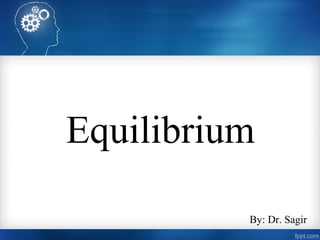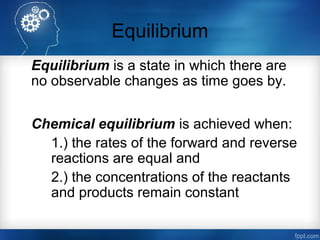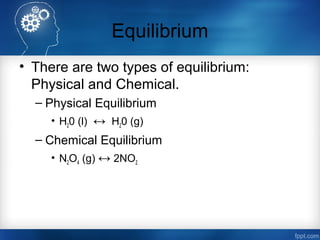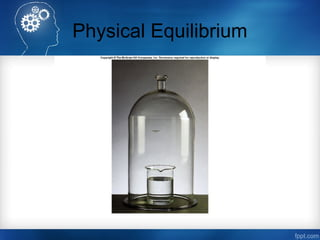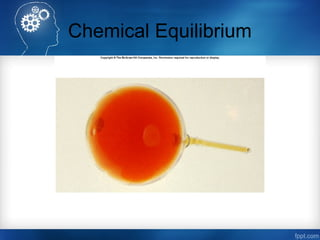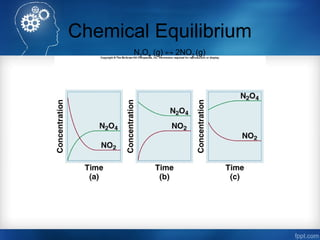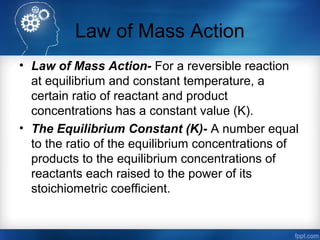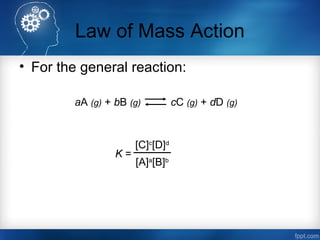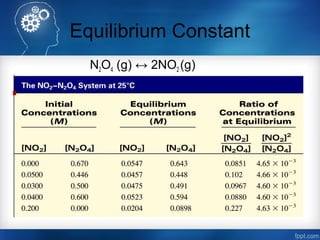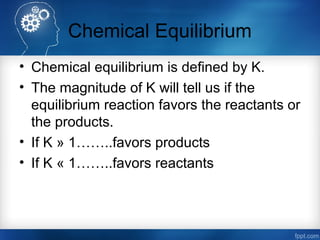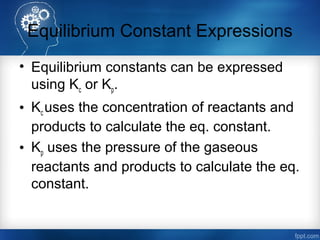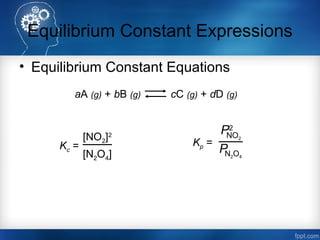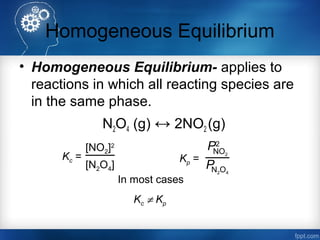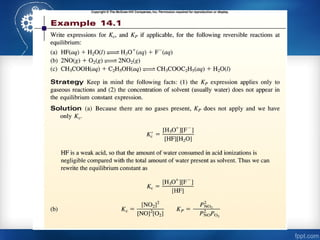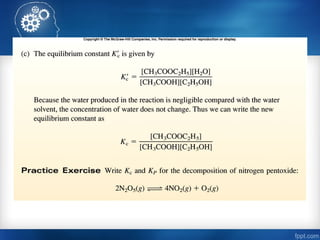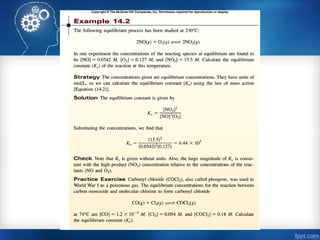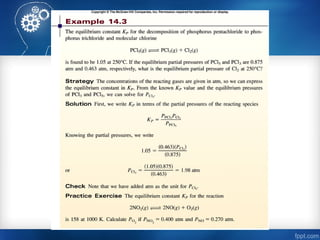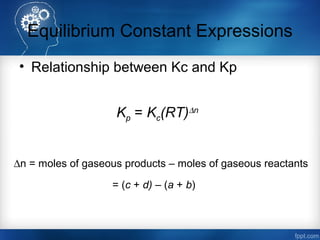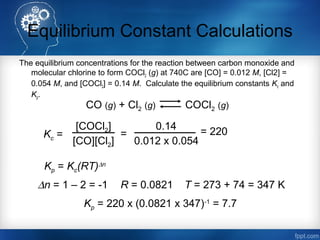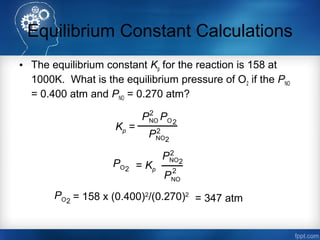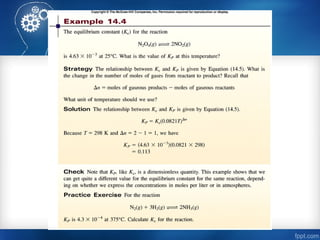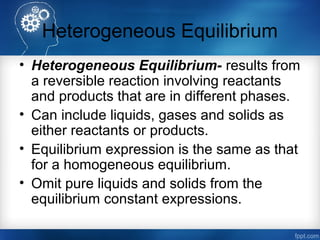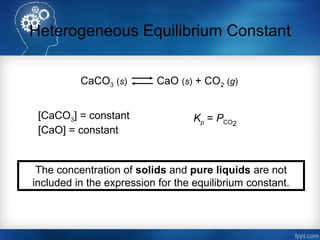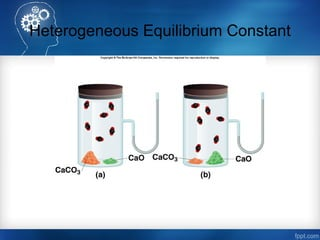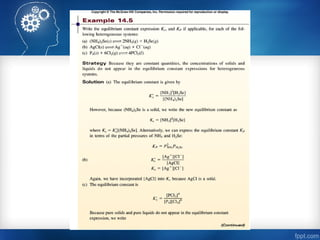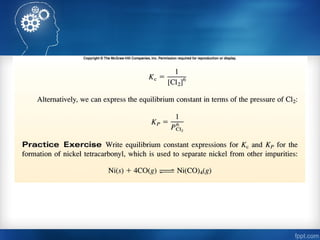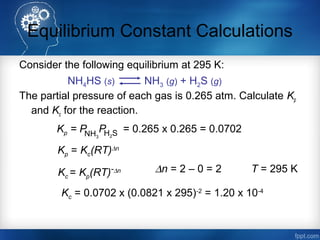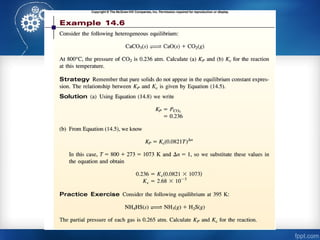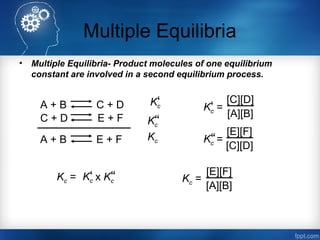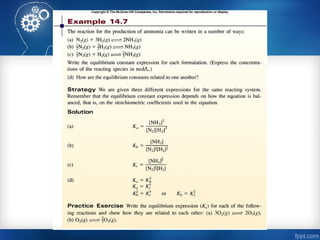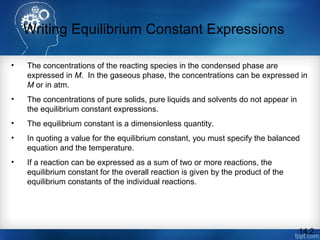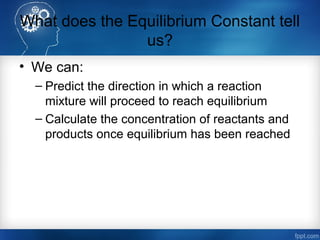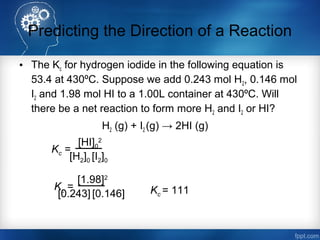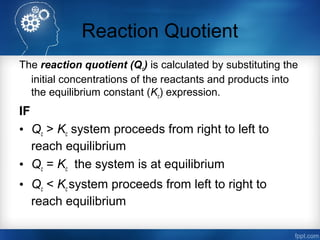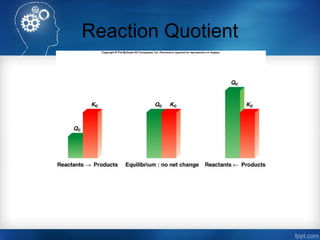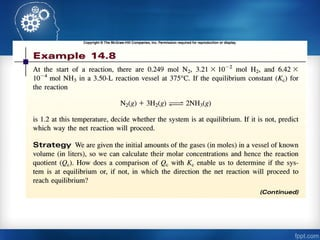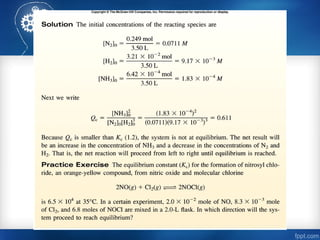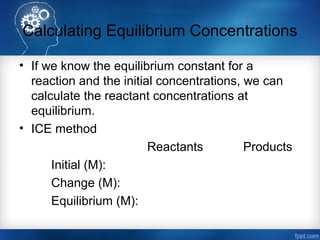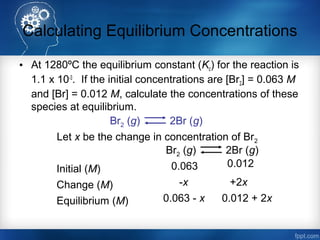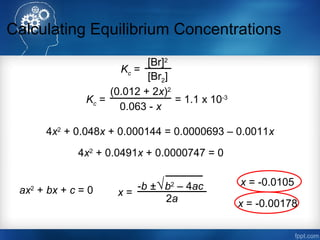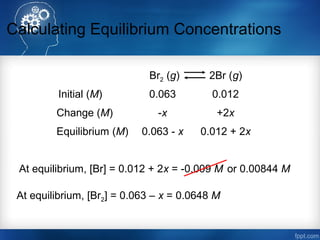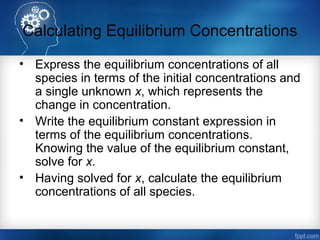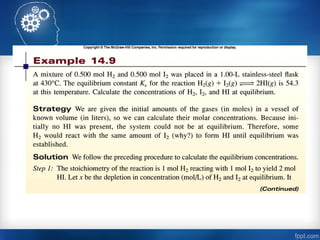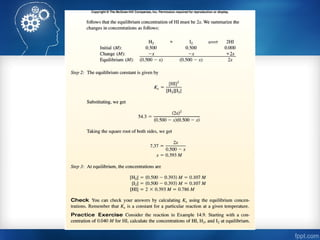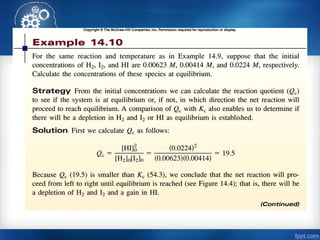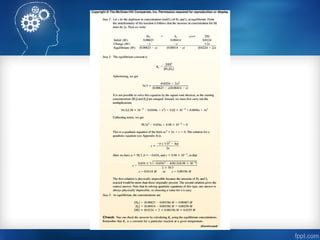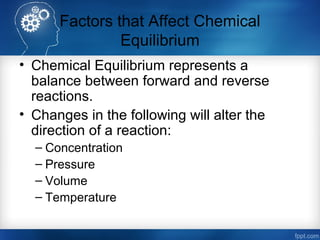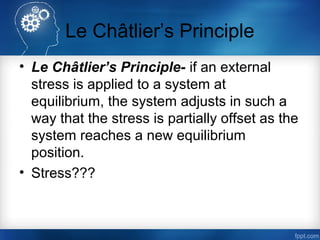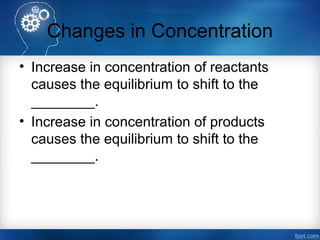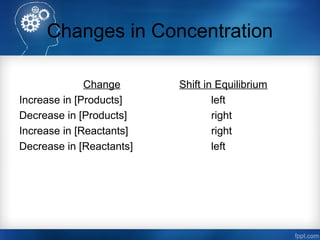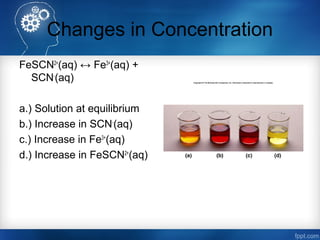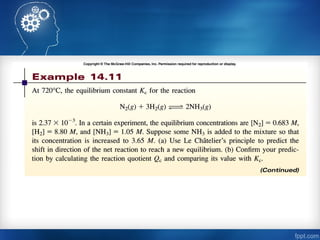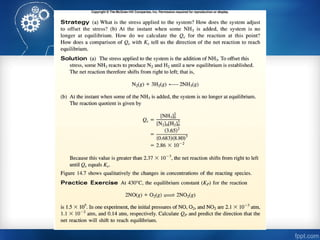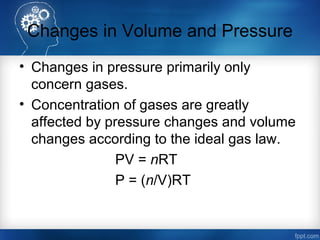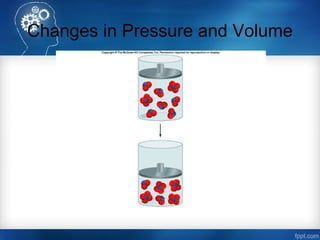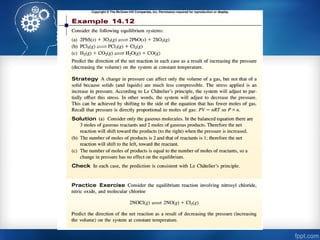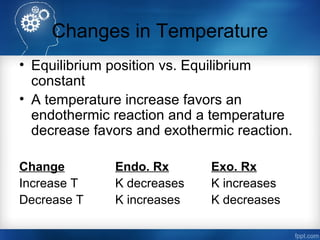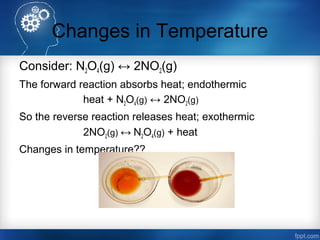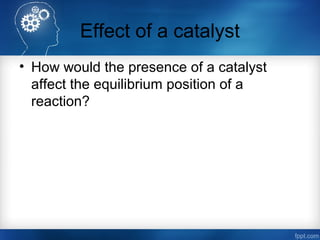1 de 60

### Chemical equilibrium

• 1. Equilibrium By: Dr. Sagir
• 2. Equilibrium Equilibrium is a state in which there are no observable changes as time goes by. Chemical equilibrium is achieved when: 1.) the rates of the forward and reverse reactions are equal and 2.) the concentrations of the reactants and products remain constant
• 3. Equilibrium • There are two types of equilibrium: Physical and Chemical. – Physical Equilibrium • H20 (l) ↔ H20 (g) – Chemical Equilibrium • N2O4 (g) ↔ 2NO2
• 4. Physical Equilibrium
• 5. Chemical Equilibrium
• 6. Chemical Equilibrium N2O4 (g) ↔ 2NO2 (g)
• 7. Law of Mass Action • Law of Mass Action- For a reversible reaction at equilibrium and constant temperature, a certain ratio of reactant and product concentrations has a constant value (K). • The Equilibrium Constant (K)- A number equal to the ratio of the equilibrium concentrations of products to the equilibrium concentrations of reactants each raised to the power of its stoichiometric coefficient.
• 8. Law of Mass Action • For the general reaction: K = [C]c [D]d [A]a [B]b aA (g) + bB (g) cC (g) + dD (g)
• 9. Equilibrium Constant N2O4 (g) ↔ 2NO2 (g)
• 10. Chemical Equilibrium • Chemical equilibrium is defined by K. • The magnitude of K will tell us if the equilibrium reaction favors the reactants or the products. • If K » 1……..favors products • If K « 1……..favors reactants
• 11. Equilibrium Constant Expressions • Equilibrium constants can be expressed using Kc or Kp. • Kc uses the concentration of reactants and products to calculate the eq. constant. • Kp uses the pressure of the gaseous reactants and products to calculate the eq. constant.
• 12. Equilibrium Constant Expressions • Equilibrium Constant Equations Kc = [NO2]2 [N2O4] Kp = NO2 P2 N2O4 P aA (g) + bB (g) cC (g) + dD (g)
• 13. Homogeneous Equilibrium • Homogeneous Equilibrium- applies to reactions in which all reacting species are in the same phase. N2O4 (g) ↔ 2NO2 (g) Kp = NO2 P2 N2O4 P In most cases Kc ≠ Kp Kc = [NO2]2 [N2O4]
• 18. Equilibrium Constant Expressions • Relationship between Kc and Kp Kp = Kc(RT)∆n ∆n = moles of gaseous products – moles of gaseous reactants = (c + d) – (a + b)
• 19. Equilibrium Constant Calculations The equilibrium concentrations for the reaction between carbon monoxide and molecular chlorine to form COCl2 (g) at 740C are [CO] = 0.012 M, [Cl2] = 0.054 M, and [COCl2] = 0.14 M. Calculate the equilibrium constants Kc and Kp. CO (g) + Cl2 (g) COCl2 (g) Kc = [COCl2] [CO][Cl2] = 0.14 0.012 x 0.054 = 220 Kp = Kc(RT)∆n ∆n = 1 – 2 = -1 R = 0.0821 T = 273 + 74 = 347 K Kp = 220 x (0.0821 x 347)-1 = 7.7
• 20. Equilibrium Constant Calculations • The equilibrium constant Kp for the reaction is 158 at 1000K. What is the equilibrium pressure of O2 if the PNO = 0.400 atm and PNO = 0.270 atm? Kp = 2 PNO PO 2 PNO 2 2 PO2 = Kp PNO 2 2 PNO 2 PO2 = 158 x (0.400)2 /(0.270)2 = 347 atm
• 22. Heterogeneous Equilibrium • Heterogeneous Equilibrium- results from a reversible reaction involving reactants and products that are in different phases. • Can include liquids, gases and solids as either reactants or products. • Equilibrium expression is the same as that for a homogeneous equilibrium. • Omit pure liquids and solids from the equilibrium constant expressions.
• 23. Heterogeneous Equilibrium Constant CaCO3 (s) CaO (s) + CO2 (g) [CaCO3] = constant [CaO] = constant Kp = PCO2 The concentration of solids and pure liquids are not included in the expression for the equilibrium constant.
• 24. Heterogeneous Equilibrium Constant
• 27. Equilibrium Constant Calculations Consider the following equilibrium at 295 K: The partial pressure of each gas is 0.265 atm. Calculate Kp and Kc for the reaction. NH4HS (s) NH3 (g) + H2S (g) Kp = PNH3 H2SP = 0.265 x 0.265 = 0.0702 Kp = Kc(RT)∆n Kc = Kp(RT)-∆n ∆n = 2 – 0 = 2 T = 295 K Kc = 0.0702 x (0.0821 x 295)-2 = 1.20 x 10-4
• 29. Multiple Equilibria • Multiple Equilibria- Product molecules of one equilibrium constant are involved in a second equilibrium process. A + B C + D C + D E + F A + B E + F Kc‘ Kc‘‘ Kc Kc = Kc‘‘Kc‘ x Kc =‘ [C][D] [A][B] Kc =‘‘ [E][F] [C][D] [E][F] [A][B] Kc =
• 31. Writing Equilibrium Constant Expressions • The concentrations of the reacting species in the condensed phase are expressed in M. In the gaseous phase, the concentrations can be expressed in M or in atm. • The concentrations of pure solids, pure liquids and solvents do not appear in the equilibrium constant expressions. • The equilibrium constant is a dimensionless quantity. • In quoting a value for the equilibrium constant, you must specify the balanced equation and the temperature. • If a reaction can be expressed as a sum of two or more reactions, the equilibrium constant for the overall reaction is given by the product of the equilibrium constants of the individual reactions. 14.2
• 32. What does the Equilibrium Constant tell us? • We can: – Predict the direction in which a reaction mixture will proceed to reach equilibrium – Calculate the concentration of reactants and products once equilibrium has been reached
• 33. Predicting the Direction of a Reaction • The Kc for hydrogen iodide in the following equation is 53.4 at 430ºC. Suppose we add 0.243 mol H2, 0.146 mol I2 and 1.98 mol HI to a 1.00L container at 430ºC. Will there be a net reaction to form more H2 and I2 or HI? H2 (g) + I2 (g) → 2HI (g) [HI]0 2 [H2]0 [I2]0 Kc = [1.98]2 [0.243] [0.146] Kc = Kc = 111
• 34. Reaction Quotient The reaction quotient (Qc) is calculated by substituting the initial concentrations of the reactants and products into the equilibrium constant (Kc) expression. IF • Qc > Kc system proceeds from right to left to reach equilibrium • Qc = Kc the system is at equilibrium • Qc < Kc system proceeds from left to right to reach equilibrium
• 35. Reaction Quotient
• 38. Calculating Equilibrium Concentrations • If we know the equilibrium constant for a reaction and the initial concentrations, we can calculate the reactant concentrations at equilibrium. • ICE method Reactants Products Initial (M): Change (M): Equilibrium (M):
• 39. Calculating Equilibrium Concentrations • At 1280ºC the equilibrium constant (Kc) for the reaction is 1.1 x 10-3 . If the initial concentrations are [Br2] = 0.063 M and [Br] = 0.012 M, calculate the concentrations of these species at equilibrium. Br2 (g) 2Br (g) Let x be the change in concentration of Br2 Br2 (g) 2Br (g) Initial (M) Change (M) Equilibrium (M) 0.063 -x 0.063 - x 0.012 +2x 0.012 + 2x
• 40. Calculating Equilibrium Concentrations [Br]2 [Br2] Kc = Kc = (0.012 + 2x)2 0.063 - x = 1.1 x 10-3 4x2 + 0.048x + 0.000144 = 0.0000693 – 0.0011x 4x2 + 0.0491x + 0.0000747 = 0 ax2 + bx + c = 0 -b ± b2 – 4ac√ 2a x = x = -0.00178 x = -0.0105
• 41. Calculating Equilibrium Concentrations Br2 (g) 2Br (g) Initial (M) Change (M) Equilibrium (M) 0.063 0.012 -x +2x 0.063 - x 0.012 + 2x At equilibrium, [Br] = 0.012 + 2x = -0.009 M At equilibrium, [Br2] = 0.063 – x = 0.0648 M or 0.00844 M
• 42. Calculating Equilibrium Concentrations • Express the equilibrium concentrations of all species in terms of the initial concentrations and a single unknown x, which represents the change in concentration. • Write the equilibrium constant expression in terms of the equilibrium concentrations. Knowing the value of the equilibrium constant, solve for x. • Having solved for x, calculate the equilibrium concentrations of all species.
• 47. Factors that Affect Chemical Equilibrium • Chemical Equilibrium represents a balance between forward and reverse reactions. • Changes in the following will alter the direction of a reaction: – Concentration – Pressure – Volume – Temperature
• 48. Le Châtlier’s Principle • Le Châtlier’s Principle- if an external stress is applied to a system at equilibrium, the system adjusts in such a way that the stress is partially offset as the system reaches a new equilibrium position. • Stress???
• 49. Changes in Concentration • Increase in concentration of reactants causes the equilibrium to shift to the ________. • Increase in concentration of products causes the equilibrium to shift to the ________.
• 50. Changes in Concentration Change Shift in Equilibrium Increase in [Products] left Decrease in [Products] right Increase in [Reactants] right Decrease in [Reactants] left
• 51. Changes in Concentration FeSCN2+ (aq) ↔ Fe3+ (aq) + SCN- (aq) a.) Solution at equilibrium b.) Increase in SCN- (aq) c.) Increase in Fe3+ (aq) d.) Increase in FeSCN2+ (aq)
• 54. Changes in Volume and Pressure • Changes in pressure primarily only concern gases. • Concentration of gases are greatly affected by pressure changes and volume changes according to the ideal gas law. PV = nRT P = (n/V)RT
• 55. Changes in Pressure and Volume Change Shift in Equilibrium Increase in Pressure Side with fewest moles Decrease in Pressure Side with most moles Increase in Volume Side with most moles Decrease in Volume Side with fewest moles
• 56. Changes in Pressure and Volume
• 58. Changes in Temperature • Equilibrium position vs. Equilibrium constant • A temperature increase favors an endothermic reaction and a temperature decrease favors and exothermic reaction. Change Endo. Rx Exo. Rx Increase T K decreases K increases Decrease T K increases K decreases
• 59. Changes in Temperature Consider: N2O4(g) ↔ 2NO2(g) The forward reaction absorbs heat; endothermic heat + N2O4(g) ↔ 2NO2(g) So the reverse reaction releases heat; exothermic 2NO2(g) ↔ N2O4(g) + heat Changes in temperature??
• 60. Effect of a catalyst • How would the presence of a catalyst affect the equilibrium position of a reaction?

### Notas del editor

1. In equilibrium, the concentration on reactants and products remains constant. Constant transfer. Although it seems like there are no more reactions taking place, r to p and p to r formation is continuously occurring. There are several types of equilibrium reactions that can occur and we will discuss all of them. In this chapter we will be looking at the types of equilibrium reactions, the equilibrium constant and its relationship to the rate constant and factors that can disrupt a system at equilibrium. Most reactions are reversible. As soon as p forms, r begins to reform. R then forms more p.
2. Physical- equilibrium between two phases of the same substance. Changes that occur are physical. Chemical- equilibrium chemists are more interested in this b/c the progress of the reaction can be easily monitored.
3. Liquid water in equilibrium with its vapor. The number of water molecules leaving the liquid is equivalent to those returning to the liquid.
4. Reversible reaction between nitrogen dioxide and dinitrogen tetroxide forms an orange colored product. Nit. Di. Is colorless and dinit. Tet. Is brown. Brown forms immediately, followed by the color lightnening due to reverse reaction occurring.
5. a.) initially only NO2 b.) Initially only N2O4 c.) Mixture Equilibrium always occurs and at app. The same time.
6. Greater than 1 = any number greater than 10 Less than 1 = any number less than 0.1
7. R = 0.0821 L x atm/K x mol
8. Liquids and solids are “1” in these problems because they are a constant. Molar concentration is density. Density never changes regardless of quantity present.
9. Partial pressure of CO2 does not change regardless of the changes in concentration of solids. Temp. has to stay the same.
10. Quote the balanced equation, temperature given from the calculation. This is because the different ways to balance the equation will result in different equilibrium values.
11. Only true for point of equilibrium.